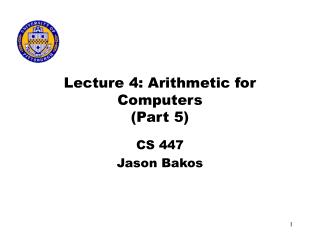# Lecture 4: Arithmetic for Computers (Part 5) - PowerPoint PPT PresentationDownload PresentationLecture 4: Arithmetic for Computers (Part 5)

Lecture 4: Arithmetic for Computers (Part 5)
Download Presentation## Lecture 4: Arithmetic for Computers (Part 5)

- - - - - - - - - - - - - - - - - - - - - - - - - - - E N D - - - - - - - - - - - - - - - - - - - - - - - - - - -
##### Presentation Transcript

1. Lecture 4: Arithmetic for Computers(Part 5) CS 447 Jason Bakos

2. Binary Division • Like last lecture, we’ll start with some basic terminology… • Again, let’s assume our numbers are base 10, but let’s only use 0’s and 1’s

3. Binary Division • Recall: • Dividend=Quotient*Divisor + Remainder • Let’s assume that both the dividend and divisor are positive and hence the quotient and the remainder are nonnegative • The division operands and both results are 32-bit values and we will ignore the sign for now

4. First Hardware Design for Divider Initialize the Quotient register to 0, initialize the left-half of the Divisor register with the divisor, and initialize the Remainder register with the dividend (right-aligned)

5. Second Hardware Design for Divider Much like with the multiplier, the divisor and ALU can be reduced to 32-bits if we shift the remainder right instead of shifting the divisor to the left Also, the algorithm must be changed so the remainder is shifted left before the subtraction takes place

6. Third Hardware Design for Divider Shift the bits of the quotient into the remainder register… Also, the last step of the algorithm is to shift the left half of the remainder right 1 bit

7. Signed Division • Simplest solution: remember the signs of the divisor and the dividend and then negate the quotient if the signs disagree • The dividend and the remainder must have the same signs

8. Considerations • The same hardware can be used for both multiply and divide • Requirement: 64-bit register that can shift left or right and a 32-bit ALU that can add or subtract

9. Floating Point • Floating point (also called real) numbers are used to represent values that are fractional or that are too big to fit in a 32-bit integer • Floating point numbers are expressed in scientific notation (base 2) and are normalized (no leading 0’s) • 1.xxxx2 * 2yyyy • In this case, xxxx is the significand and yyyy is the exponent

10. Floating Point • In MIPS, a floating point is represented in the following manner (IEEE 754 standard): • bit 31: sign of significand • bit 30..23 (8) exponent (2’s comp) • bit 22..0 (23) significand • Note that size of exponent and significand must be traded off... accuracy vs. range • This allows us representation for signed numbers as small as 2x10-38 to 2x1038 • Overflow and underflow must be detected • Double-precision floating point numbers are 2 words... the significand is extended to 52 bits and the exponent to 11 bits • Also, the first bit of the significand is implicit (only the fractional part is specified) • In order to represent 0 in a float, put 0 in the exponent field • So here’s the equation we use: (-1)S x (1+Significand) x 2E • Or: (-1)S X (1+ (s1x2-1) + (s2x2-2) + (s3x2-3) + (s4x2-4) + ...) x 2E

11. Considerations • IEEE 754 sought to make floating-point numbers easier to sort • sign is first bit • exponent comes first • But we want an all-0 (+1) exponent to represent the most-negative exponent and an all-1 exponent to be the most positive • This is called biased-notation, so we’ll use the following equation: • (-1)S x (1 + Significand) x 2(Exponent-Bias) • Bias is 127 for single-precision and 1023 for double-precision